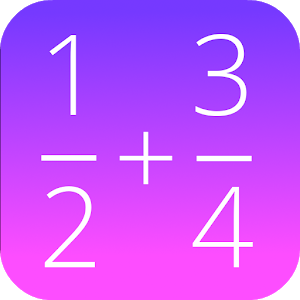Português

Todos os dias nós oferecemos aplicativos e jogos para Android licenciados GRATUITAMENTE que, caso contrário, você teria que comprar.\$0.99

# Android Giveaway of the Day - Fractions Math Pro

This math app is a fractions calculator with various functions!
avaliação do usuário: 6 (100%) 0 (0%)

This giveaway offer has been expired. Fractions Math Pro is now available on the regular basis.

This calculator is the pro version of 'Fractions Math', and completely without advertisement.

This math app is a fractions calculator with various functions:

- add, subtract, multiply and divide fractions.
- convert fractions in decimals,
- simplify fractions
- compare fractions
- find the lowest common denominator
- fraction trainer, to learn fraction calculations

GK Apps

Education

4.0

279k

### Avaliação:

Everyone